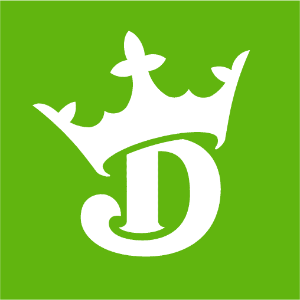DraftKings 2019 Stanley Cup Odds
+800
8 to 1Bruins
8.9% implied probability

+1000
10 to 1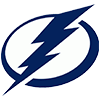Lightning
7.3% implied probability

+1300
13 to 1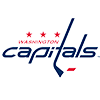Capitals
5.7% implied probability

+1300
13 to 1Avalanche
5.7% implied probability

+1300
13 to 1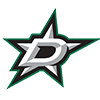Stars
5.7% implied probability

+1300
13 to 1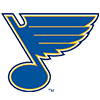Blues
5.7% implied probability

+1500
15 to 1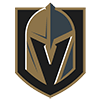Golden Knights
5.0% implied probability

+1600
16 to 1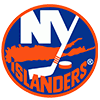Islanders
4.7% implied probability

+1600
16 to 1Predators
4.7% implied probability

+1700
17 to 1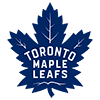Maple Leafs
4.4% implied probability

+1700
17 to 1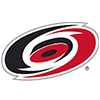Hurricanes
4.4% implied probability

+1800
18 to 1Penguins
4.2% implied probability

+1900
19 to 1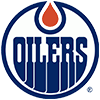Oilers
4.0% implied probability

+2300
23 to 1Coyotes
3.3% implied probability

+2600
26 to 1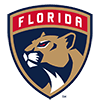Panthers
3.0% implied probability

+2700
27 to 1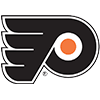Flyers
2.9% implied probability

+3000
30 to 1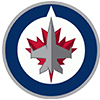Jets
2.6% implied probability

+3200
32 to 1Canucks
2.4% implied probability

+3200
32 to 1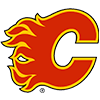Flames
2.4% implied probability

+3500
35 to 1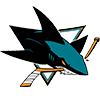Sharks
2.2% implied probability

+4000
40 to 1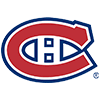Canadiens
2.0% implied probability

+5500
55 to 1Wild
1.4% implied probability

+6000
60 to 1Ducks
1.3% implied probability

+6500
65 to 1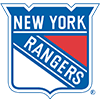Rangers
1.2% implied probability

+7000
70 to 1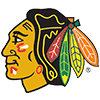Blackhawks
1.1% implied probability

+8000
80 to 1Sabres
1.0% implied probability

+9000
90 to 1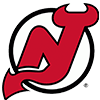Devils
0.9% implied probability

+9000
90 to 1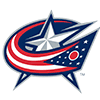Blue Jackets
0.9% implied probability

+20000
200 to 1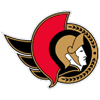Senators
0.4% implied probability

+22500
225 to 1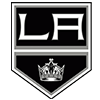Kings
0.4% implied probability

+65000
650 to 1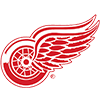Red Wings
0.1% implied probability